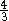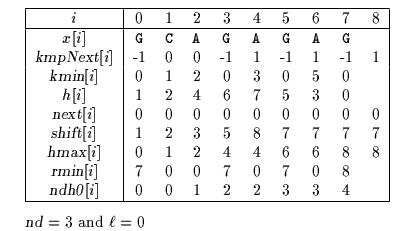Next: Apostolico-Crochemore algorithm Up: ESMAJ Prev: Colussi algorithm

# Galil-Giancarlo algorithm

Main features
• refinement of Colussi algorithm;
• preprocessing phase in O(m) time and space complexity;
• searching phase in O(n) time complexity;
• performsn text character comparisons in the worst case.
Description

The Galil-Giancarlo algorithm is a variant of the Colussi algorithm. The change intervenes in the searching phase. The method applies when x is not a power of a single character. Thus xcm with c in.

Letbe the last index in the pattern such that fori, x=x[i] and xx[+1]. Assume that during the previous attempt all the noholes have been matched and a suffix of the pattern has been matched meaning that after the corresponding shift a prefix of the pattern will still match a part of the text. Thus the window is positioned on the text factor y[j .. j+m-1] and the portion y[j .. last] matches x[0 .. last-j]. Then during the next attempt the algorithm will scanned the text character beginning with y[last+1] until either the end of the text is reached or a character xy[j+k] is found.

In this latter case two subcases can arise:x[+1]y[j+k] or too less x have been found (k) then the window is shifted and positioned on the text factor y[k+1 .. k+m], the scanning of the text is resumed (as in the Colussi algorithm) with the first nohole and the memorized prefix of the pattern is the empty word.x[+1]=y[j+k] and enough of x has been found (k >) then the window is shifted and positioned on the text factor y[k--1 .. k-+m-2], the scanning of the text is resumed (as in the Colussi algorithm) with the second nohole (x[+1] is the first one) and the memorized prefix of the pattern is x[0 ..+1].

The preprocessing phase is exactly the same as in the Colussi algorithm and can be done in O(m) space and time.

The searching phase can then be done in O(n) time complexity and furthermore at mostn text character comparisons are performed during the searching phase.

The C code
```void GG(char *x, int m, char *y, int n) {
int i, j, k, ell, last, nd;
int h[XSIZE], next[XSIZE], shift[XSIZE];
char heavy;

for (ell = 0; x[ell] == x[ell + 1]; ell++);
if (ell == m - 1)
/* Searching for a power of a single character */
for (j = ell = 0; j < n; ++j)
if (x == y[j]) {
++ell;
if (ell >= m)
OUTPUT(j - m + 1);
}
else
ell = 0;
else {
/* Preprocessing */
nd = preCOLUSSI(x, m, h, next, shift);

/* Searching */
i = j = heavy = 0;
last = -1;
while (j <= n - m) {
if (heavy && i == 0) {
k = last - j + 1;
while (x == y[j + k])
k++;
if (k <= ell || x[ell + 1] != y[j + k]) {
i = 0;
j += (k + 1);
last = j - 1;
}
else {
i = 1;
last = j + k;
j = last - (ell + 1);
}
heavy = 0;
}
else {
while (i < m && last < j + h[i] &&
x[h[i]] == y[j + h[i]])
++i;
if (i >= m || last >= j + h[i]) {
OUTPUT(j);
i = m;
}
if (i > nd)
last = j + m - 1;
j += shift[i];
i = next[i];
}
heavy = (j > last ? 0 : 1);
}
}
}

```
The example

Preprocessing phaseTables used by Galil-Giancarlo algorithm

Searching phase

References
• BRESLAUER, D., 1992, Efficient String Algorithmics, Ph. D. Thesis, Report CU-024-92, Computer Science Department, Columbia University, New York, NY.
• GALIL Z., GIANCARLO R., 1992, On the exact complexity of string matching: upper bounds, SIAM Journal on Computing, 21(3):407-437.Next: Apostolico-Crochemore algorithm Up: ESMAJ Prev: Colussi algorithm

e-mails: {Christian.Charras, Thierry.Lecroq }@laposte.net
Tue Jan 14 15:03:31 MET 1997